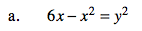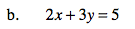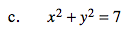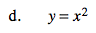### Home > PC > Chapter 11 > Lesson 11.1.3 > Problem11-44

11-44.
1. Transform the following equations from rectangular form to polar form. Homework Help ✎

1. 6x − x2 = y2

2. 2x + 3y = 5

3. x2 + y2 = 7

4. y = x2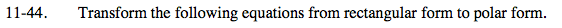Recall:
x = r cos(θ)
y = r sin(θ)
x2 + y2 = r2